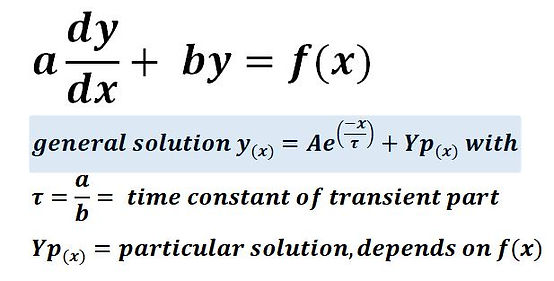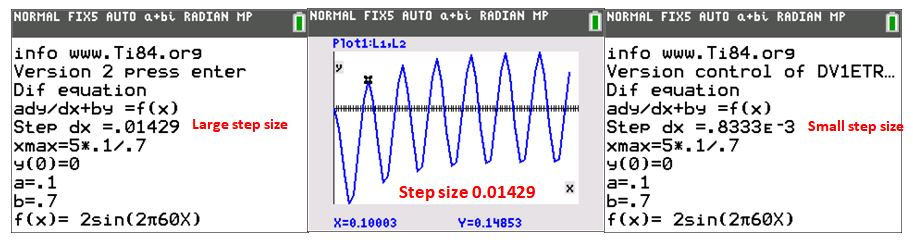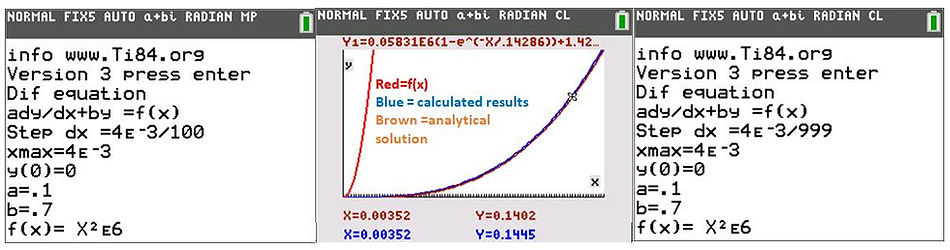naver-site-verification: naver47238a1b6bfbb19a2fd4b619734fa9a6.html
top of page

# More about the step size choice

## Get insight into the step size choice for the numerical programs of Ti-84 plus

### 1: How to select the appropriate step size for solving first order differential equations solved with the Ti-84 plus

Gaining insight into the numerical processes is import for electrical engineers who apply numerical programs such as Simulink and Multisim during their work.

In the numerical programs written for the Ti-84 plus CE calculator, such as the first order differential equation solver DV1ETRP3.8XP, a step size must also be chosen before the program starts calculations. A good choice of the step size is important because if the step size is too small, then calculation time takes a long time and memory overflow can occur. If the step size is too large, then the results are no longer reliable. In the figure below, the calculated results with a good step size and with a wrong step size are shown. If a wrong step size is chosen, then calculated results are incorrect and the plot may appear inaccurate. However, it is not always so simple to determine that the results are incorrect. Using practical examples, we will clarify what is a good choice for the step size and not through complicated theories. Also, a method for controlling the reliability and accuracy of the calculated results will be explained

To understand the explanation, knowledge of solving first-order differential equations analytically is necessary. Insight into the effects of step size on the accuracy of first order differential equation solver results is also useful for other numerical solvers written for the Ti calculator, such as second order differential equation solver or numerical integrals. Let us start with researching the step size for linear first order differential equation solvers. The general equation of a linear first order differential equation Is :To obtain good numerical results, the step size  must be at least ten times smaller than the time constant. For a constant or slowly changing f(x), the  requirements related to the time constant are sufficient.

Suppose a=0.1, b=0.7 and f(x)=2(constant). The step size choice is 0.1/(10*.7)=0.01429 sec. Comparing the results of the numerical calculations with the analytical solution shows that the program gives good results with this rather large step size.  A fast changing f(x) however, sets stricter requirements on the step size. We will demonstrate this with the following example.Suppose that the input signal f(x)= 2 sin(2π60x) instead of a constant f(x)=2, and we use the same time step as before = 0.01429 sec., then the program produces wrong results. As the frequency of the input is 60 Hz, which is a period of time of 0.0166 sec and almost equals the step size, it is clear that no reliable results can be obtained.A smaller step size is necessary. From experience 20 samples of a sinusoidal input signal and trapezoidal solver are sufficient to get reliable results which means that the step size is decreased to T/20= 1/(f*20) =1/(60*20)= 0.0008333 sec.

This is 17.15 times smaller than the time used at constant f(x) as input. Here below, the results calculated with a large step size (blue) and with a small step size (red) are shown. The results totally differ. The red results are OK.For other signals than sinusoidal signals, we advise  taking a step size equal to Xmax/999.

For example, we take f(x)= (x^2)/1E-6 in the area 0<x<4E-3. Xmax=4E-3.

Below, you can see the difference in results between a step size of Xmax/100 and Xmax/999

The program DV1ETRP3 can save up to 999 points, so a step size of 4E-3/999= 4.004E-6 gives 999   calculated points.

A step size 10 times smaller shows that the calculated and simulated results agree goodSummarizing the rules for the step size for a first order differential equation :

1: determine the time constant a/b. The step size should be a/(10b) for slow-changing function f(x).

2: For rapidly changing f(x) a step size of Xmax/100 is usually sufficient but can be chosen smaller. The maximum of saved points is 999. Then the step size is Xmax/999. A smaller step size is possible, but be aware that only 999 points will be saved.

2: How to select the appropriate step size for second order differential equations solved with the Ti-84Too large a step size gives inaccurate results with second order differential solver as well. Similar rules for the step size for the second order differential equation solver can be derived.

1: Calculate dx =  (√(A/C)) /20 and dx =(B/C)/10 and take the smallest step size of both.

2 For rapidly changing f(x), take a step size of dx= Xmax/999. To get confidence in the results, you can repeat the calculations with a step size of dx= Xmax/2000. If the results between both are small (less than 4 %) than you can trust the results.

The maximum of saved points is 999. Then the step size = Xmax/999. A smaller step size is possible, but be aware that only 999 points are saved.

bottom of page